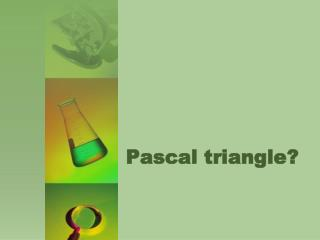DownloadDownload PresentationPascal triangle ?

# Pascal triangle ?

Download Presentation## Pascal triangle ?

- - - - - - - - - - - - - - - - - - - - - - - - - - - E N D - - - - - - - - - - - - - - - - - - - - - - - - - - -
##### Presentation Transcript

1. Pascal triangle?

2. Blaise Pascal (Blaise Pascal) was born 1623, in Clermont, France. His father, whowas educated chose not to study mathematics before the 15th year. In the 12th year, Blaze was decided to teach geometry to discover that the interior angles of a triangle is equal to twice the right corner. Pascal has never married because of his decision to devote himself to science. After a series of health problems, Pascal umro1662. in his 39th year. In 1968, a programming language, PASCAL, is named after Blaise Pascal.

3. Pascal's triangle is also named after Blaise Pascal, because a lot of mathematical formulas involved with Pascal's triangle, and it was just one of his many projects. The first written records of Pascal's triangle come yet from the Indian mathematician Pingala, 460 years before the new eregde spomenje and the Fibonacci sequence, and the sum of the diagonals of Pascal triangle.

4. Otherwise, Pascal's triangle was discovered by a Chinese mathematician, Chu Shu-Kie 1303rd year.The Figure shows originali record such a triangle.

5. The construction of Pascal triangle • At the beginning, at the top of the triangle, the zero type of the registration number 1 The first type is to write two units. In each type the first number is 1 and can be smatratiti as the sum of 1 +0. Further, the basic idea consists in the fact that we add two numbers above (from one species), and obtained a sum we write in the box (the middle), below or to the next race. These steps are repeated until the order is filled, then the process is repeated on a new line. Line 0 0 1 0 + + Line 1 1 1 0 0 + + + Line 2 0 1 2 1 0 + + + + Line 3… 1 3 3 1 tn,r = tn-1,r-1 + tn-1,r

6. Dividing cells How to order meal? Coins Natural numbers Binomial formula Polygonal numbers Serpinski triangle Points on the circle Number11 Coloring

7. Fibonacci sequence Fibonacci sequence is given by recurrent formula , and its terms are given by: Fibonacci sequence can be vizualiyed by using package GeoGebraasFibonacijev1.ggb

8. The raising of rabbits and Fibonaci sequence A man buys a couple of rabbits and raised their offspring in the following order:Parental pair of rabbits is marked with number 1. 1pair After the first month a couple of young rabbits (first generation) is born, which is also marked with 1 1 new pair After the second month the parental pair get another pair and then the parents breaks on reproduction, but a couple of rabbits from the first generation also gets some new rabits. The number of pairs is now 22 pairs

9. The raising of rabbits and Fibonaci sequence This mode of reproduction is true for all the descendants of the parental couple. Each new pair of rabbits will be given in two consecutive months a pair of young rabits,and after that its propagation will be terminated. In the fourth month the pair of new born obtain: a pair of first-generation and second generation of two pairs, so the number of pairs is 3. 3pairs In the fifth month will be 5 pairs of rabbits, 5pairs in the sixth month will be 8pairs of rabbits, and so on. • 8pairs

10. The raising of rabbits and Fibonaci sequence The number of pairs of rabbits after each month corresponds to Fibonacci series. We can say that after half a year we have 21 pair of rabbits, and after one year 233 pairs of rabbits Rabbits

11. If we sum up the numbers on the diagonals of Pascal triangle then we get a series Fibonacije numbers.

12. If the term of Fibonacci sequence is divided with the following term of Fibonacci sequence, then the following sequence is obtained: 1/1 1/2 2/3 3/5 5/8 8/13 13/21 21/34 34/55 55/89 .……. The Fibonacci sequence diverges, but the sequence of Fabonacci quotients of terms, converges to the golden section.

13. Fibonacci sequence in nature can be seen on the website: http://britton.disted.camosun.bc.ca/fibslide/jbfibslide.htm. In her presentation Irina Boyadjiev, as can be seen on the website showed the Fibonacci spirals in nature: http://www.lima.ohio-state.edu/people/iboyadzhiev/GeoGebra/spiralInNature.html Analogously the authors made: FibonacijevS.ggbFibonacijevSK1.ggbFibonacijevSL.ggb